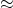Essential Concept 5: Problems in Regression Analysis | IFT World
101 Concepts for the Level I Exam

# Essential Concept 5: Problems in Regression AnalysisProblem Effect Solution
Heteroskedasticity: variance of error term is not constant.
Test using BP test
BP = nRF-test is unreliable. Standard error underestimated.
t-stat overstated.
Robust standard errors
Generalized least squares
Serial correlation: error terms are correlated. Test using DW stat.
DW2(1 – r)
F-stat too high. Standard error underestimated.
t-stat overstated.
Hansen method
Modify the regression equation
Multicollinearity: two or more independent variables are highly correlated. Inflated SE’s; t-stats of coefficients artificially small
High ROmit one or more of the independent variables

LIVE WEEKEND CLASSES *For 2024 Exam candidates*
This is default text for notification bar